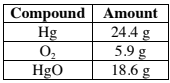# Problem: Mercury and oxygen react to form mercury(II) oxide as shown below:2 Hg(l) + O2(g) ⇌ 2 HgO(s)At a certain temperature in a 8.4 L reaction vessel that has reached equilibrium, the chemical components have the following composition:Which of the following is true if the mass of all the chemical components double and the volume remains constant? (Hg: 48.8 g, O2: 11.8 g, HgO: 37.2 g)A. Q &gt; K, shift left B. Q &gt; K, shift right C. Q &lt; K, shift leftD. Q &lt; K, shift right E. Q = K, no shift

###### FREE Expert Solution

We are asked to calculate the Q and K values and determine the shift in the reaction.

The formula for Q is:

The formula for K is:

Notice that K only differs from Q in a way that the concentration or pressures must be at equilibrium for K but not for Q.

Depending on if Q is greater than or less than K, our reaction will shift to attain equilibrium by reaching the equilibrium constant K:

If Q = K           → the reaction is at equilibrium

If Q < K           → the reaction shifts in the forward direction to reach equilibrium

If Q > K           → the reaction shifts in the reverse direction to reach equilibrium

Take note that we don't include solids or liquids in the K and Q expressions.

This means that in the problem:

2 Hg(l) + O2(g) ⇌ 2 HgO(s)

We are only concerned with O2.

We go through the following steps to solve the problem:

Step 1. Calculate the molar mass O2

Step 2. Calculate the molarity O2 for both scenarios.

Step 3. Calculate Q and K.

Step 4. Compare Q and K.

92% (235 ratings)###### Problem Details

Mercury and oxygen react to form mercury(II) oxide as shown below:

2 Hg(l) + O2(g) ⇌ 2 HgO(s)

At a certain temperature in a 8.4 L reaction vessel that has reached equilibrium, the chemical components have the following composition:Which of the following is true if the mass of all the chemical components double and the volume remains constant? (Hg: 48.8 g, O2: 11.8 g, HgO: 37.2 g)

A. Q > K, shift left

B. Q > K, shift right

C. Q < K, shift left

D. Q < K, shift right

E. Q = K, no shift